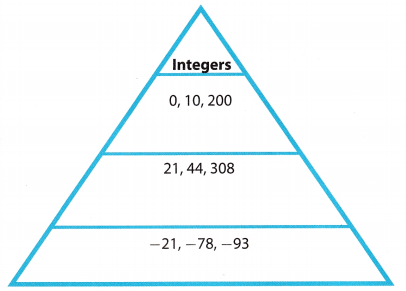# Texas Go Math Grade 8 Module 1 Answer Key Real Numbers

Refer to our Texas Go Math Grade 8 Answer Key Pdf to score good marks in the exams. Test yourself by practicing the problems from Texas Go Math Grade 8 Module 1 Answer Key Real Numbers.

## Texas Go Math Grade 8 Module 1 Answer Key Real Numbers

Essential Question
How cart you use real numbers to solve real-world problems?Complete these exercises to review the skills you will need for this chapter.

Find the Square of a Number

Example
Find the Square of $$\frac{2}{3}$$Find the square of each number.

Go Math Grade 8 Module 1 Question 1.
7 _____
72 Given
7 * 7 Multiply the number by itself
49 Simplify
49

Question 2.
21 _____
212 Given
21 * 21 Multiply the number by itself
441 Simplify
441

Question 3.
-3
(-3)2 Given
(-3) * (-3) Multiply the number by itself
9 Simplify
9

$$\frac{4}{5}$$ ____
$$\left(\frac{4}{5}\right)^{2}$$ Given
$$\frac{4}{5}$$ * $$\frac{4}{5}$$
$$\frac{4 * 4}{5 * 5}$$
$$\frac{16}{25}$$ Simplify
$$\frac{16}{25}$$

Question 5.
2.7 ________
2.72 Given
2.7 * 2.7 Multiply the number by itself
7.29 Simplify
7.29

Question 6.
–$$\frac{1}{4}$$ ________
Multiply the number by itself:
$$\left(-\frac{1}{4}\right)$$ × $$\left(-\frac{1}{4}\right)$$ = $$\frac{(-1) \times(-1)}{4 \times 4}$$
$$\frac{1}{16}$$

Question 7.
-5.7 _______
(-5.7)2 Given
(-5.7) * (-5.7) Multiply the number by itself
32.49 Simplify
32.49

Question 8.
1$$\frac{2}{5}$$ ____
$$\left(1 \frac{2}{5}\right)^{2}$$ Given
$$\left(\frac{7}{5}\right)^{2}$$ Rewrite as improper fraction
$$\left(\frac{7}{5}\right)$$ * $$\left(\frac{7}{5}\right)$$ Multiply the number by itself
$$\frac{7 * 7}{5 * 5}$$
$$\frac{49}{25}$$ Simplify
1$$\frac{24}{25}$$

Exponents
Example 53 = 5 × 5 × 5 Use the base, 5, as a factor 3 times.
= 25 × 5 Multiply from left to right
= 125

Simplify each exponential expression.

Question 9.
92 _________
92 Given
9 * 9 Use the base 9 as a factor for 2 times
81 Multiply from left to right
81

Question 10.
24 __________
24 Given
2 * 2 * 2 * 2 Use the base 2 as a factor for 4 times

4 * 2 * 2 Multiply from left to right
8 * 2
16

($$\frac{1}{3}$$)2
Use the base $$\frac{1}{3}$$ as a factor 2 times:
($$\frac{1}{3}$$)2 = $$\frac{1}{3}$$ × $$\frac{1}{3}$$
Multiply from left to right:
$$\frac{1}{9}$$

Question 12.
(-7)2 _______
(-7)2 Given
(-7) * (-7) Use the base -7 as a factor for 2 times
49 Multiply from left to right
49

Question 13.
43 _________
43 Given
4 * 4 * 4 Use the base 4 as a factor for 3 times

16 * 4 Multiply from left to right
64

Question 14.
(-1)5 ______
Use the base -1 as a factor 5 times:
(-1)5 = (-1) × (-1) × (-1) × (-1) × (-1)

Multiply from left to right:
(-1)5 = (-1) × (-1) × (-1) × (-1) × (-1)
= 1 × (-1) × (-1) × (-1)
= (-1) × (-1) × (-1)
= 1 ×(-1)
= -1

Algebra 1 Module 1 Answer Key Question 15.
4.52 _______
4.52 Given
(4.5) * (4.5) Use the base 4.5 as a factor for 2 times
20.25 Multiply from left to right

Question 16.
105 ________
105 Given
1o * 10 * 10 * 10 * 10 Use the base 10 as a factor for 5 times
1oo * 10 * 10 * 10 Multiply from left to right
1000 * 10 * 10
10000 * 10
100000

Write each mixed number as an improper fraction.

Question 17.
3$$\frac{1}{3}$$ _______
3$$\frac{1}{3}$$ Given
3 + $$\frac{1}{3}$$ Write the mixed number as a sum of whole number and a fraction

$$\frac{9}{3}$$ + $$\frac{1}{3}$$ Write the whole number as an equivalent fraction with the same denominator as the fraction
$$\frac{10}{3}$$ Add the numerators
$$\frac{10}{3}$$

Question 18.
1$$\frac{5}{8}$$ _______
1$$\frac{5}{8}$$ Given
1 + $$\frac{5}{8}$$ Write the mixed number as a sum of whole number and a fraction
$$\frac{8}{8}$$ + $$\frac{5}{8}$$ Write the whole number as an equivalent fraction with the same denominator as the fraction
$$\frac{13}{8}$$ Add the numerators
$$\frac{13}{8}$$

2$$\frac{3}{7}$$
2$$\frac{3}{7}$$ Given
2 + $$\frac{3}{7}$$
Write the mixed number as a sum of a whole number and a fraction
$$\frac{14}{7}$$ + $$\frac{3}{7}$$ Write the whole number as an equivalent fraction with the same denominator as the fraction
$$\frac{17}{7}$$ Add the numerators
$$\frac{17}{7}$$

Question 20.
5$$\frac{5}{6}$$ _______
5$$\frac{5}{6}$$ Given
5 + $$\frac{5}{6}$$ Write the mixed number as a sum of whole number and a fraction
$$\frac{30}{6}$$ + $$\frac{5}{6}$$ Write the whole number as an equivalent fraction with
the same denominator as the fraction
$$\frac{35}{6}$$ Add the numerators
$$\frac{35}{6}$$

Visualize Vocabulary

Use the ✓ words to complete the graphic. You can put more than one word In each section of the triangle.Understand Vocabulary

Complete the sentences using the preview words.

Question 1.
One of the two equal factors of a number is a ____